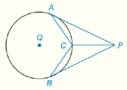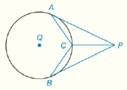Chapter 6.CR, Problem 29CR### Elementary Geometry for College St...

6th Edition
Daniel C. Alexander + 1 other
ISBN: 9781285195698

#### Solutions

Chapter
Section### Elementary Geometry for College St...

6th Edition
Daniel C. Alexander + 1 other
ISBN: 9781285195698
Textbook Problem
1 views

# In Review Exercises 27 to 29, give a proof for each statement. G i v e n :                 A P -   a n d   B P -   a r e   t a n g e n t   t o   ⊙ Q   a t   A   a n d   B                                                               C   i s   t h e   m i d p o i n t   o f   A B ^             P r o v e :                     P C →   b i s e c t s   ∠ A P BTo determine

To Prove: PC bisects APB, if AP- and BP- are tangent to Q at A and B. C is the midpoint of AB^.Explanation

Concept:

Theorem 6.1.3:

In a circle (or in congruent circles), congruent minor arcs have congruent central angles.

Theorem 6.1.3:

In a circle (or in congruent circles), congruent central angles have congruent minor arcs.

Theorem 6.1.5:

In a circle (or in congruent circles), congruent chords have congruent minor (major) arcs.

Theorem 6.1.6:

In a circle (or in congruent circles), congruent arcs have congruent chords.

Theorem 6.1.7:

Chords that are at the same distance from the center of a circle are congruent.

Theorem 6.1.8:

Congruent chords are located at the same distance from the center of a circle.

Theorem 6.2.4:

The measure of an angle formed by a tangent and a chord drawn to the point of tangency is one-half the measure of the intercepted arc.

Theorem 6.3.4:

The tangent segments to a circle from an external point are congruent.

Calculation:

Given AP- and BP- are tangent to Q at A and B. C is the midpoint of AB^.

By Theorem 6.3.4:

The tangent segments to a circle from an external point are congruent.

So, AP=BP

Since, C is the midpoint of AB^.

So, AC^=CB^

By, Theorem 6.1.6:

In a circle (or in congruent circles), congruent arcs have congruent chords.

Because congruent chords, AC=CB.

Theorem 6.1.3:

In a circle (or in congruent circles), congruent minor arcs have congruent central angles.

Since, AC^=CB^ then AQC=BQC

By Theorem 6

### Still sussing out bartleby?

Check out a sample textbook solution.

See a sample solution

#### The Solution to Your Study Problems

Bartleby provides explanations to thousands of textbook problems written by our experts, many with advanced degrees!

Get Started

#### Find each limit. a. limx2| x2 |x2 b. limx2+| x2 |x2

Calculus: An Applied Approach (MindTap Course List)

#### Simplify: 1240

Elementary Technical Mathematics

#### Express the function in the form f g h. 51. S(t) = sin2(cos t)

Single Variable Calculus: Early Transcendentals, Volume I

#### The polar form for the graph at the right is:

Study Guide for Stewart's Multivariable Calculus, 8th

#### True or False: x2x24dx should be evaluated using integration by parts.

Study Guide for Stewart's Single Variable Calculus: Early Transcendentals, 8th

#### Define the concept of external validity and a threat to external validity.

Research Methods for the Behavioral Sciences (MindTap Course List)